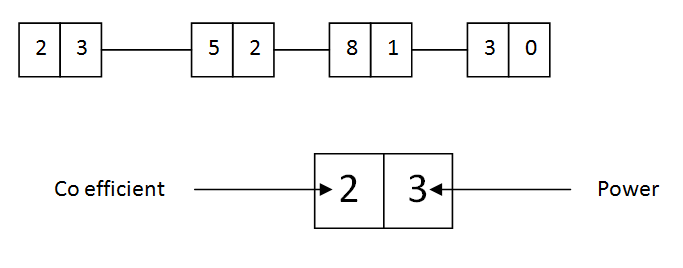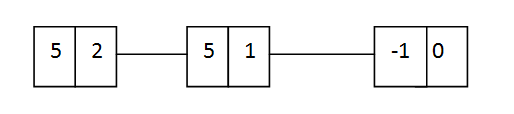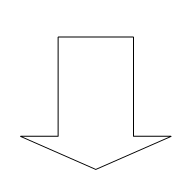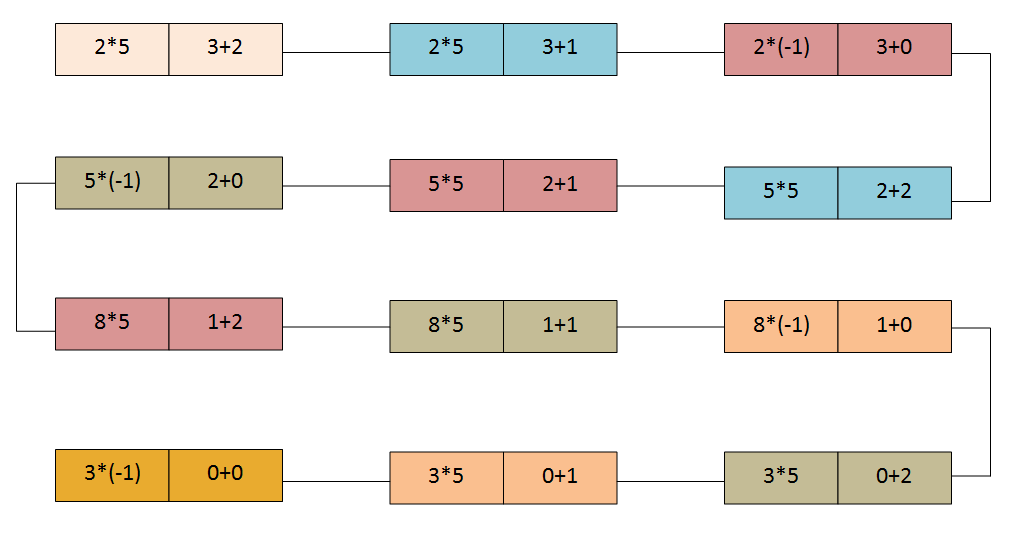# Polynomials

Consider following polynomials.

1. 2x3 + 5x2+8x+3
2. 5x2+5x-1

This is how the first polynomial is represented using nodes.This is how the second is represented using nodes.The node consists of 2 parts. First part has the coefficient and the second part consists of the power of the variable.

Adding above polynomials is as following.Coefficients of same powered nodes are added together, powers are not added and they remain same.

## Multiplication of polynomials

Multiplication of above polynomials is as following.

Each and every node of the first polynomial is multiplied with each and every node of the second polynomial.When multiplying, the coefficients are multiplied and the powers are added. Then nodes with the same powers are added.Created By:

#### Thilini Cooray

Edited and Posted By:

#### Praneeth Nilanga Peiris

• අනාථ මාරුතේ , Direct link to comment

nicely composed in an understandable way… RocK ON !!!!

• PARIZ , Direct link to comment

Thanks machn..

• , Direct link to comment

elaaazz…thanx…

• , Direct link to comment

thanks buddy, i’m trying to code it… but it seems to difficult to handle.. so much conditions.. it’s nice if you can code it.. cheers 😀